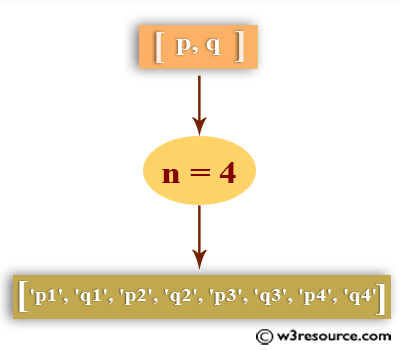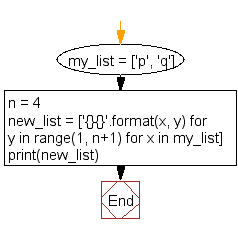﻿ Python: Create a list by concatenating a given list which range goes from 1 to n - w3resource# Python: Create a list by concatenating a given list which range goes from 1 to n

## Python List: Exercise - 35 with Solution

Write a Python program to create a list by concatenating a given list which range goes from 1 to n.Sample Solution:-

Python Code:

``````my_list = ['p', 'q']
n = 4
new_list = ['{}{}'.format(x, y) for y in range(1, n+1) for x in my_list]
print(new_list)
```
```

Sample Output:

```['p1', 'q1', 'p2', 'q2', 'p3', 'q3', 'p4', 'q4']
```

Flowchart:## Visualize Python code execution:

The following tool visualize what the computer is doing step-by-step as it executes the said program:

Python Code Editor:

Have another way to solve this solution? Contribute your code (and comments) through Disqus.

What is the difficulty level of this exercise?

Test your Programming skills with w3resource's quiz.

﻿

## Python: Tips of the Day

Invokes the provided function after ms milliseconds:

Example:

```from time import sleep

def tips_delay(fn, ms, *args):
sleep(ms / 1000)
return fn(*args)
print(tips_delay(
lambda x: print(x),
1000,
'w3r'
))
```

Output:

```w3r
None
```